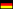Hamburg University of Technology / Institute of Mathematics / Research Topics / Stokes Operator on Lipschitz DomainsStokes Operator on Lipschitz Domains

Description

In the solution theory for nonlinear partial differential equations, an integral part of the solution process is often to develop a semigroup theory for the linearization of the equation. In the case of the famous Navier-Stokes equations which for a given domain $$\Omega \subseteq \mathbb{R}^d$$, $$d \geq 2$$, describe the behavior of a Newtonian fluid over time, the linearization is given by the Stokes equations

$\partial_t u - \Delta u + \nabla \pi = 0 \quad\text{in } \Omega\,, \;t > 0\,, \quad \operatorname{div}(u) = 0 \quad\text{in } \Omega\,,\; t > 0\,,$

$u(0) = a \text{ in } \Omega\,, u = 0 \text{ on } \partial\Omega\,,\; t > 0\,,$

where $$u \colon \mathbb{R}^+ \times \Omega \to \mathbb{R}^d$$ stands for the velocity field and $$\pi \colon \mathbb{R}^+ \times \Omega \to \mathbb{R}$$ represents the pressure of the fluid. The so-called Stokes semigroup $$(\mathrm{e}^{-tA})_{t \geq 0}$$ describes the evolution of the velocity $$u$$ and the Stokes operator $$A$$ corresponds to the term ??$$-\Delta u + \nabla \pi$$?? in the Stokes equations.

Having a semigroup makes it possible to look for mild solutions to the Navier-Stokes equations using a variation of constants formula to construct an iteration method. This approach was introduced by Fujita and Kato  and builds mainly on resolvent estimates for the Stokes operator $$A$$ and the analyticity property of the Stokes semigroup.

 Fujita, H. and Kato, T. On the Navier-Stokes initial value problem I. Archive for Rational Mechanics and Analysis 16(1964), 269?315.

 Tolksdorf, P. On the Lp-theory of the Navier-Stokes equations on Lipschitz domains. PhD thesis, Technische Universität Darmstadt, 2017. Available at http://tuprints.ulb.tu-darmstadt.de/5960/.

 Gabel, F. On Resolvent Estimates in Lp for the Stokes Operator in Lipschitz Domains. Master thesis, Technische Universität Darmstadt, 2018.

 Gabel, F. and Tolksdorf, P. The Stokes operator in two-dimensional bounded Lipschitz domains. In preparation.